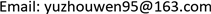1. 引言

2. 问题描述

min ∑ i = 0 n l i s .t . f i ≤ ∑ i = 0 n p i , ∀ i s i + p i = f i , ∀ i (1)

3. 调度算法

1) 某种顺序的任务排列： { t 0 , t 1 , ⋯ , t k − 1 , t k + 1 , ⋯ , t k + δ } ；

2) 任务 t k ；

3) 某种顺序的任务排列： { t k + δ + 1 , t k + δ + 2 , ⋯ , t n } ；

f k ( δ ) = ∑ j ≤ k | δ p j (2)

V ( ∅ , γ ) = 0 (3)

V ( { j } , γ ) = max ( 0 , γ + p j − d j ) (4)

V ( T ( j , n , k ) , t ) = min ( V ( j , k ′ + δ , k ′ ) , γ ) + max ( 0 , f k ′ ( δ ) − d k ′ )     + V ( T ( k ′ + δ + 1 , n , k ′ ) , f k ′ ( δ ) ) (5)

p k ′ = max ( p j ′ | j ′ ∈ T ( j , n , k ) ) (6)

V ( { t 0 , t 1 , ⋯ , t n } , 0 ) (7)

4. 激励机制

C i = α p i ⋅ β d i − p i ⋅ e γ P (8)

5. 实验与性能分析

5.1. 实验设置

5.2. 结果分析

6. 结语